# VLOOKUP function in Excel

The VLOOKUP function will automatically find the value you need from any list. In this article you'll see how to use the VLOOKUP function and how to easily avoid the mistakes that most people make when it comes to using the VLOOKUP function in a formula. Steps are given below.

##### Formula:

VLOOKUP (lookup_value, table_array, col_index_num, [range_lookup])

Step 1: First create two sheet “Price List” and “Sales Order” as below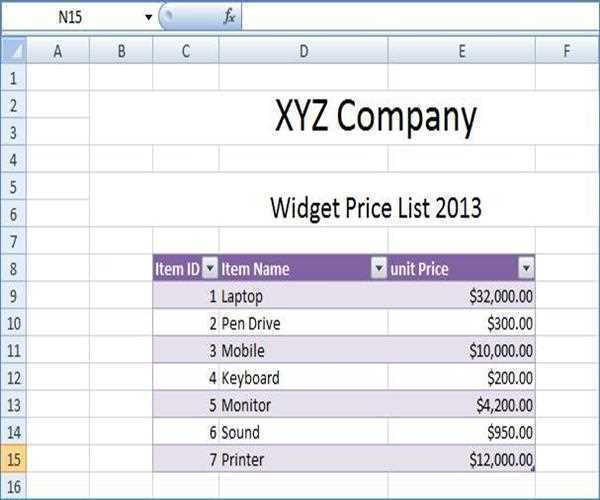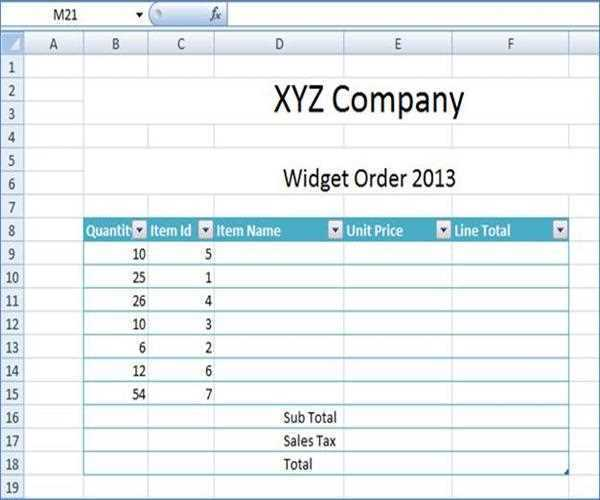Step 2: Now select cell on “Sales Order” where we apply VLOOKUP formula and give lookup_value index as below image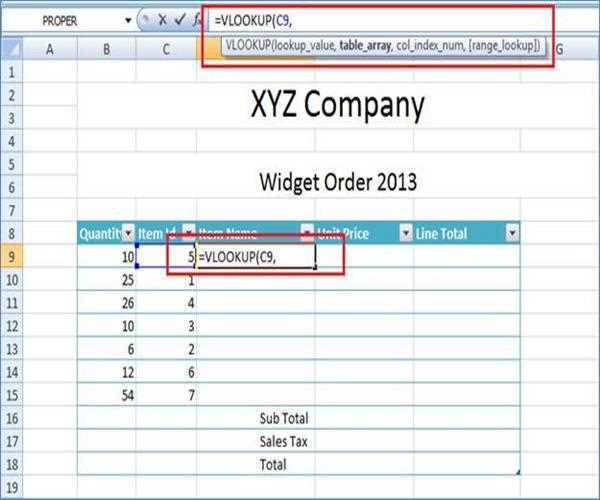Now give the table_array value as below image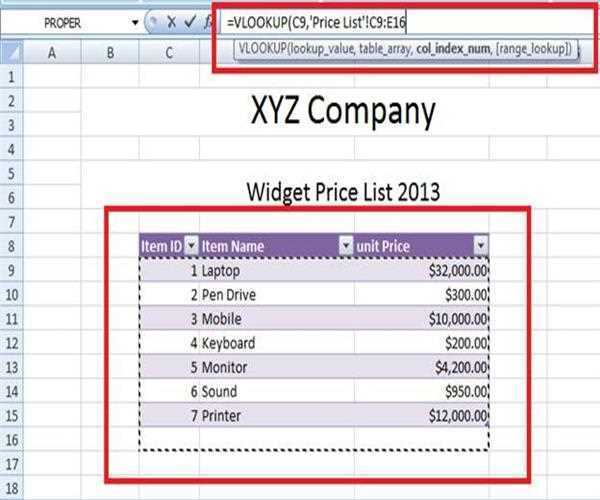Put the comma after table_array parameter and give the col_index_num. Here col_index_num  is 2, and set [range_lookup] as false. Hence whole formula is

=VLOOKUP (C9,'Price List'!C9:E16,2,FALSE)

Now press enter button and see output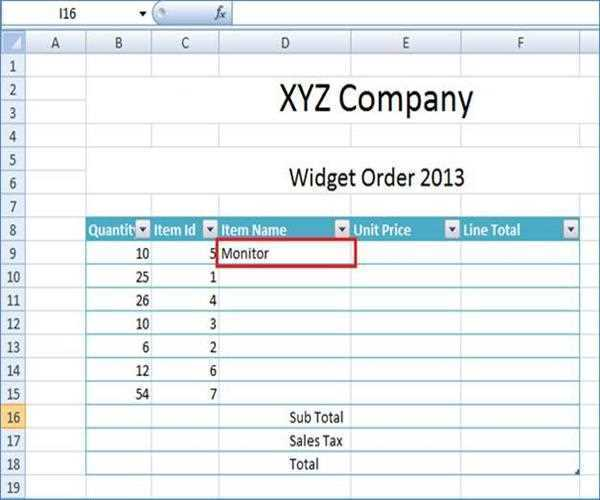Step 3: Now apply all cell of column “Item Name”.

Step 4: Apply same process for display “Unit Price” as below image, formula for unit price as following

=VLOOKUP (C9,'Price List'!C9:E16,3,FALSE)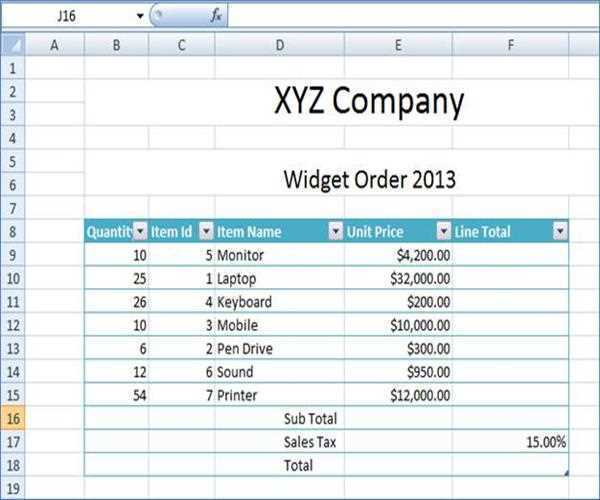Step 5: Now you can calculate “Total” amount and percentage and whole output screen as below. Get the help for calculating sum, product and percentage from following link.

http://www.mindstick.com/Articles/e790a001-fcca-4077-bdbe-66da92bcc5fb/?Sum%20Function%20in%20Excel

http://www.mindstick.com/Articles/2820228e-d10a-438e-808d-00879cce50a3/?How%20to%20do%20percentages%20in%20Excel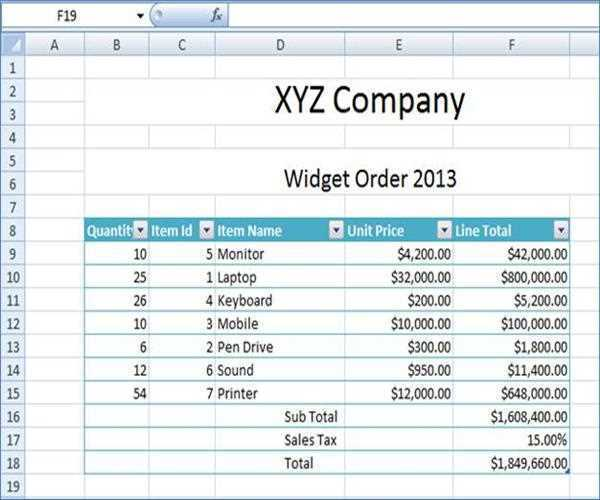Last updated:9/7/2019 12:14:10 AMAvadhesh Kumar Patel District Project Manager - Aligarh 14 months work experience in Panchayati Raj Department Sector as District Project Manager & 12 months work experience in IT Sector as Software Engineer. :-)##### Rajdip Sarkar

Dear Sir,

I have one scenario in "V-Lookup", I'm unable to doing, what would be the solution.
Sheet 1Sheet 2If I want to change any value from Sheet 1 automatically reflect "Sheet1" Total row & "Sheet 2 "Total row.